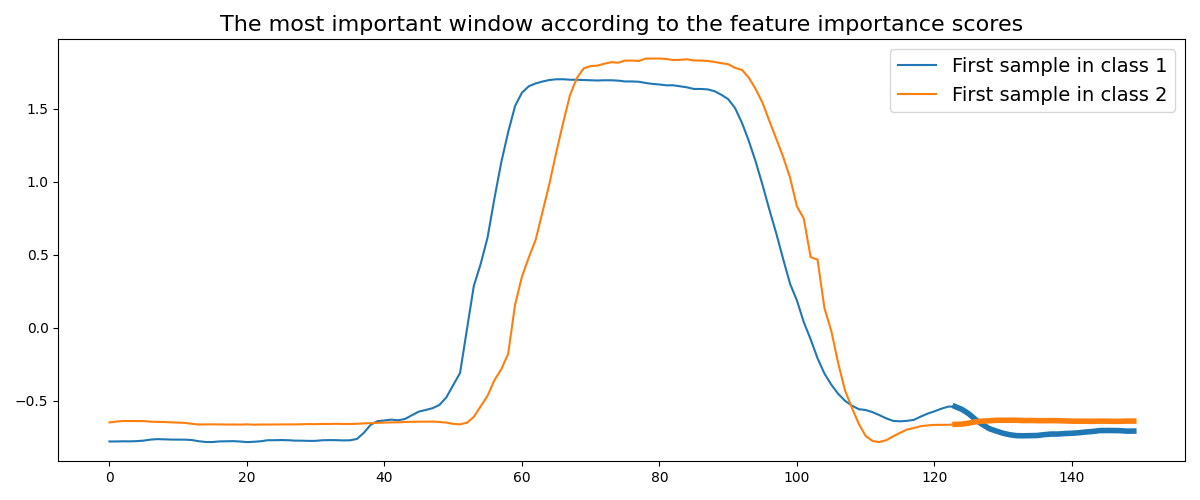# Time Series Forest¶

This example illustrates which information is considered important by the algorithm in order to classify time series. The index of the most important window is retrieved via the `feature_importance_` and `indices_` attributes. The first time series for both classes are plotted and the most important window is highlighted with a larger line width.

It is implemented as `pyts.classification.TimeSeriesForest`.```# Author: Johann Faouzi <johann.faouzi@gmail.com>

import numpy as np
from pyts.classification import TimeSeriesForest
import matplotlib.pyplot as plt

X_train, X_test, y_train, y_test = load_gunpoint(return_X_y=True)
clf = TimeSeriesForest(random_state=43)
clf.fit(X_train, y_train)

start_idxmax, end_idxmax = clf.indices_[
np.argmax(clf.feature_importances_) // 3]

plt.figure(figsize=(12, 5))
plt.plot(X_train[y_train == 1], label='First sample in class 1')
plt.plot(np.arange(start_idxmax, end_idxmax),
X_train[y_train == 1][0, start_idxmax:end_idxmax],
color='C0', lw=4)

plt.plot(X_train[y_train == 2], label='First sample in class 2')
plt.plot(np.arange(start_idxmax, end_idxmax),
X_train[y_train == 2][0, start_idxmax:end_idxmax],
color='C1', lw=4)

plt.legend(loc='best', fontsize=14)
plt.title('The most important window according to the feature importance '
'scores', fontsize=16)
plt.tight_layout()
plt.show()
```

Total running time of the script: ( 0 minutes 1.733 seconds)

Gallery generated by Sphinx-Gallery